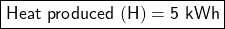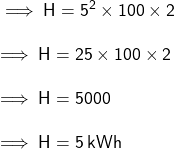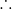## a current of 5 ampere is passed for 2 hours in an electric iron having a resistance of 100 ohms calculate the heat produced

Question

a current of 5 ampere is passed for 2 hours in an electric iron having a resistance of 100 ohms calculate the heat produced

in progress 0
2 months 2021-07-30T22:05:32+00:00 1 Answers 3 views 0Given:

Resistance (R) = 100 Ω

Current (I) = 5 A

Time (t) = 2 hours

To Find:

Heat developed (H) in the electric iron

Explanation:

Formula:Substituting values of I, R & t in the equation:Heat developed (H) in the electric iron = 15 kWh